# 1 Friday, July 30

References:

Stated goal of the course: prove that the moduli space $$\mkern 1.5mu\overline{\mkern-1.5mu{ \mathcal{M}_{g} }\mkern-1.5mu}\mkern 1.5mu$$ of stable curves (for $$g\geq 2$$) is a smooth, proper, irreducible Deligne-Mumford stack of dimension $$3g-3$$. Moreover, it admits a projective coarse moduli space.

In the process we’ll define algebraic spaces and stacks.

Prerequisites:

• Schemes
• Existence of Hilbert schemes
• Artin approximation
• Resolution of singularities for surfaces
• Deformation theory

# 2 Lecture 3: Groupoids and Prestacks (Monday, September 06)

## 2.1 Groupoids

Last time: functors, sheaves on sites, descent, and Artin approximation. Today: groupoids and stacks.

Recall that a site $$\mathsf{S}$$ is a category such that for all $$U\in {\operatorname{Ob}}(\mathsf{S})$$, there exists a set $${\mathsf{Cov}}(U) \mathrel{\vcenter{:}}=\left\{{U_i \to U}\right\}_{i\in I}$$ (a covering family) such that

• $$\operatorname{id}_U \in {\mathsf{Cov}}(U)$$,
• $${\mathsf{Cov}}(U)$$ is closed under composition.
• $${\mathsf{Cov}}(U)$$ is closed under pullbacks: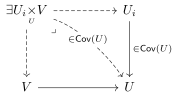Take $$\mathsf{S} \mathrel{\vcenter{:}}={\mathsf{Sch}}_{\text{Ét}}$$ to be the big étale site: the category of all schemes, with covering families given by étale morphisms $$\left\{{U_i\to U}\right\}_{i\in I}$$ such that $$\displaystyle\coprod_i U_i \twoheadrightarrow U$$. Note that there is a special covering family given by surjective etale morphisms.

Let $$\mathsf{C}$$ be a category (e.g. $$\mathsf{C} \mathrel{\vcenter{:}}={\mathsf{Set}}$$) and recall that a presheaf on a category $$\mathsf{S}$$ is a contravariant functor $$\mathsf{S}\to \mathsf{C}$$.

A $$\mathsf{C}{\hbox{-}}$$valued sheaf on a site $$\mathsf{S}$$ is a presheaf \begin{align*} {\mathcal{F}}:\mathsf{S} \to \mathsf{C} \end{align*} such that for all $$U_i, U_j\in {\mathsf{Cov}}(U)$$, the following equalizer diagram is exact in $$\mathsf{C}$$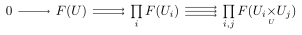Show that a presheaf $$F$$ is a sheaf on $${\mathsf{Sch}}_\text{Ét}$$ iff

• $$F$$ is a sheaf on $${\mathsf{Sch}}_{\mathrm{Zar}}$$ and
• For all etale surjections $$U' \twoheadrightarrow_{\text{ét}} U$$ of affines, the equalizer diagram is exact.

For $$X\in {\mathsf{Sch}}$$, the presheaf \begin{align*} h_X \mathrel{\vcenter{:}}=\operatorname{Mor}({-}, X): {\mathsf{Sch}}\to {\mathsf{Set}} \end{align*} is a sheaf on $${\mathsf{Sch}}_{\text{Ét}}$$.

We’ll often consider moduli functors: functors $$F: {\mathsf{Sch}}\to {\mathsf{Set}}$$ where $$F(S)$$ is a family of objects over $$S$$. Then $$F$$ will be a sheaf iff families glue uniquely in the étale topology, and representability of such functors will imply they are sheaves.

Consider the following moduli functor:This is not representable by a scheme and not a sheaf.

Why care about representability? Suppose there were a scheme $$M$$, so \begin{align*} F_{{\mathsf{Alg}}}(S) \simeq \operatorname{Mor}(S, M) .\end{align*} Then taking $$\operatorname{id}_M \in \operatorname{Mor}(M, M)$$ should yield a universal family $${\mathcal{U}}\to M$$: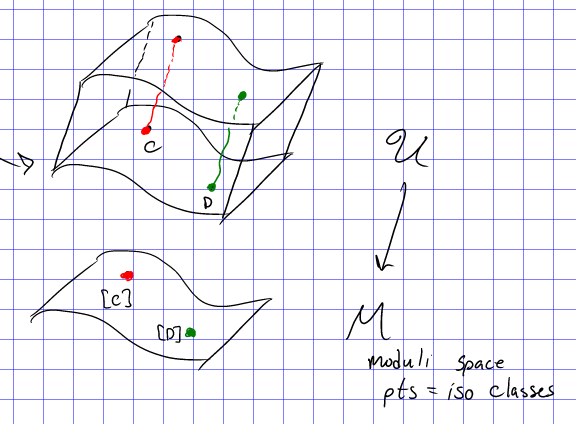Then the points of $$M$$ would correspond to isomorphism classes of curves, and every family of curves would be a pullback of this.

For any $$S\in{\mathsf{Sch}}$$ and a family $${\mathcal{C}}\xrightarrow{f} S$$, the fiber $$f^{-1}(s)\in{\mathcal{C}}$$ is a curve for any $$s\in S$$, so one could define a map \begin{align*} g: S &\to M \\ s &\mapsto [s] ,\end{align*} where we send a curve to its isomorphism class. Then $${\mathcal{C}}$$ would fit into a pullback diagram:If $$S$$ was itself a curve, then $$g: S\to M$$ would be a path in $$M$$ deforming a base curve.

## 2.2 Groupoids

Recall that a groupoid is a category where every morphism is an isomorphism. Morphisms of groupoids are functors, and isomorphisms of groupoids are equivalences of categories.

A basic example is the category of sets where \begin{align*} \operatorname{Mor}(A, B) \mathrel{\vcenter{:}}= \begin{cases} \operatorname{id}_A & A=B \\ \emptyset & \text{else}. \end{cases} \end{align*}

A similar construction: for any set $$\Sigma$$, one can form a groupoid $${\mathcal{C}}_\Sigma$$:

• Object: Elements $$x\in \Sigma$$.
• Morphisms: $$\operatorname{id}_x$$

Define a category $${ \mathcal{M}_{g} }({\mathbb{C}})$$:

• Objects: smooth projective curves over $${\mathbb{C}}$$ of genus $$g$$.
• Morphisms: \begin{align*} \operatorname{Mor}(C, C') = \mathop{\mathrm{Isom}}_{{\mathsf{Sch}}_{/ {{\mathbb{C}}}} }(C, C') \subseteq \operatorname{Mor}_{{\mathsf{Sch}}_{/ {{\mathbb{C}}}} }(C, C') .\end{align*}

Groupoids are equivalent iff they are equivalent as categories. The following is an example of mapping the quotient groupoid $$[C_2/C_4]$$ to $${\mathsf{B}}C_2$$: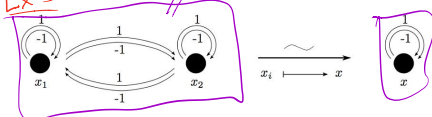If a groupoid $${\mathfrak{X}}$$ is equivalent to $$\mathsf{C}_{\Sigma}$$ for any $$\Sigma \in {\mathsf{Set}}$$, we say $${\mathfrak{X}}$$ is equivalent to a set. For example, the following groupoid is equivalent to a 2-element set: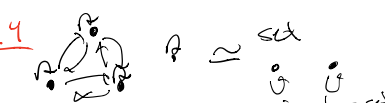For $$G\curvearrowright\Sigma$$ a group acting on any set, define the quotient groupoid $$[\Sigma/G]$$ in the following way:

• Objects: $$x\in \Sigma$$, i.e. one object for each element of the set $$\Sigma$$.
• Morphisms: $$\operatorname{Mor}(x, x') = \left\{{g\in G {~\mathrel{\Big|}~}gx' = x}\right\}$$.

Show that $$[\Sigma/G]$$ is equivalent to a set iff $$G\curvearrowright\Sigma$$ is a free action.

For $$\Sigma = \left\{{{\operatorname{pt}}}\right\}$$, we obtain \begin{align*} {\mathsf{B}}G \mathrel{\vcenter{:}}=[{\operatorname{pt}}/ G] ,\end{align*} where there is one object $${\operatorname{pt}}$$ and $$\operatorname{Mor}({\operatorname{pt}}, {\operatorname{pt}}) = G$$.

Define $${\mathsf{FinSet}}$$ to be the category of finite sets where the morphisms are set bijections. Then $${\mathsf{FinSet}}= \displaystyle\coprod_{n\in {\mathbb{Z}}_{\geq 0}} {\mathsf{B}}S_n$$ for $$S_n$$ the symmetric group.

For $$C, D' \to D$$ morphisms of groupoids, we can construct their fiber product as the cartesian diagram: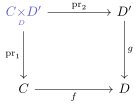It can be constructed as the following category:

\begin{align*} {\operatorname{Ob}}(C{ \underset{\scriptscriptstyle {D} }{\times} } D') \mathrel{\vcenter{:}}= \left\{ \begin{array}{l} (c, d', \alpha) \end{array} \middle\vert \begin{array}{l} c\in C, d'\in D', \\ \\ \alpha: f(c) \xrightarrow{\sim} g(d') \end{array} \right\} \end{align*}

Describe the universal property of the pullback in the 2-category of groupoids.

$$G$$ regarded as a groupoid is the pullback over inclusions of points into $${\mathsf{B}}G$$: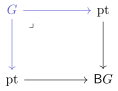Let $$G\curvearrowright\Sigma$$ and $$x\in \Sigma$$, and let $$Gx$$ be the orbit and $$G_x$$ be the stabilizer. Then there is a morphism of groupoids $$f \in \operatorname{Mor}({\mathsf{B}}G_x, [\Sigma/G])$$ inducing a pullback: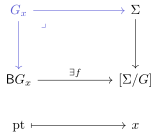## 2.3 Prestacks

Motivation: to specify a moduli functor, we’ll need the data of

• Families over $$S$$,
• How to pull back families under morphisms, and
• How objects are isomorphic.

As a first attempt, we might try to define a 2-functor $$F: {\mathsf{Sch}}\to {\mathsf{Grpd}}$$ between 2-categories, where the latter is the category of groupoids. For this, we need the following data:

• For all $$S\in {\mathsf{Sch}}$$, an assignment of a groupoid $$F(S)$$,
• For all morphisms $$f\in \operatorname{Mor}_{{\mathsf{Sch}}}(S, T)$$, an assignment of morphisms of groupoids \begin{align*} f^* \in \operatorname{Mor}_{{\mathsf{Grpd}}}(F(T), F(S)) .\end{align*}
• For compositions of morphisms of schemes $$S \xrightarrow{f} T \xrightarrow{g} U$$, an isomorphism of functors \begin{align*} \psi_{fg}: g^* \circ f^* \xrightarrow{\sim} (g \circ f)^* .\end{align*}
• Compatibility of these isomorphisms on chains of compositions $$S \to T \to U \to V \to \cdots$$.1

This is a lot of data to track, so instead we’ll construct a large category $${\mathfrak{X}}$$ that encodes all of this, along with a fibrationHere $$S \in {\mathsf{Sch}}$$ and $$F(S) \in {\mathsf{Grpd}}$$, so the “fibers” above $$S$$ are groupoids.

Let $$p:{\mathfrak{X}}\to \mathsf{C}$$ be a functor between two 1-categories, so we have the following data:Then $${\mathfrak{X}}, p$$ define a prestack over $$\mathsf{C}$$ iff

• Pullbacks exist: for $$S \xrightarrow{f} T$$, there exists a (not necessarily unique) map $$f^*b$$, sometimes denoted $${ \left.{{b}} \right|_{{f}} }$$, yielding a cartesian square: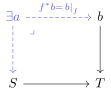• A universal property making $${\mathfrak{X}}$$ a fibered category: every arrow in $${\mathfrak{X}}$$ is a pullback, so there are always lifts of the following form: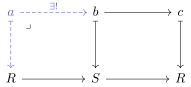An alternative definition: a prestack is a category fibered in groupoids.

We often conflate $${\mathfrak{X}}$$ and the functor $${\mathfrak{X}}\xrightarrow{p} S$$, and don’t spell out the composition law in $${\mathfrak{X}}$$. Moreover, we write $$f^*b$$ or $${ \left.{{b}} \right|_{{f}} }$$ for a choice of a pullback.

For $$p: {\mathfrak{X}}\to \mathsf{C}$$ a functor and $$S\in {\operatorname{Ob}}(\mathsf{C})$$ any fixed object, the associated fiber category over $$S$$, denoted $${\mathfrak{X}}(S)$$, is the subcategory of $${\mathfrak{X}}$$ defined by:

• Objects: $$a\in {\operatorname{Ob}}({\mathfrak{X}})$$ such that $$a \xrightarrow{p} S$$,
• Morphisms: $$\operatorname{Mor}(a, a')$$ are morphisms $$f\in \operatorname{Mor}_{{\mathfrak{X}}}(a, a')$$ over $$\operatorname{id}_S$$:We can now equivalently define presheaves as categories fibered in sets.

Show that if $${\mathfrak{X}}\to \mathsf{C}$$ is a prestack, then for all $$S\in \mathsf{C}$$, all maps in $${\mathfrak{X}}(S)$$ are invertible. Conclude that the fiber categories $${\mathfrak{X}}(S)$$ are all groupoids.

Every presheaf forms a prestack. Let $$F \in \underset{ \mathsf{pre} } {\mathsf{Sh} }({\mathsf{Sch}}, {\mathsf{Set}})$$ be a presheaf of sets, and define $${\mathfrak{X}}_F$$ as the following category:

• Objects: Pairs $$(S, a \in F(S))$$ where $$S\in {\mathsf{Sch}}$$ and $$F(s) \in {\mathsf{Set}}$$.
• Morphisms: \begin{align*} \operatorname{Mor}( (S, a), (T, b) ) \mathrel{\vcenter{:}}=\left\{{ S \xrightarrow{f} T {~\mathrel{\Big|}~}a = f^* b}\right\} .\end{align*}

Note that we’ll often conflate $$F$$ and $${\mathfrak{X}}_F$$. This yields the fibrationFor $$X\in {\mathsf{Sch}}$$, take its Yoneda functor $$h_X: {\mathsf{Sch}}\to {\mathsf{Set}}$$. Then define the category $${\mathfrak{X}}_X$$:

• Objects: Morphisms $$S\to X$$ of schemes.
• Morphisms: $$\operatorname{Mor}(S\to X, T\to X)$$ are morphisms over $$X$$: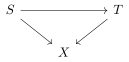This yields the fibrationDefine $${ \mathcal{M}_{g} }$$ as the following category:

• Objects: families $${\mathcal{C}}\to S$$ of smooth genus $$g$$ curves,
• Morphisms: $$\operatorname{Mor}({\mathcal{C}}\to S, {\mathcal{C}}'\to S')$$: cartesian squaresThis yields a fibrationFor $$C$$ a smooth connected projective curve over $$k$$ a field, define $${\mathsf{Bun}}(C)$$ as the following category:

• Objects: pairs $$(S, F)$$ where $$F$$ is a vector bundle over $$C\times S$$.
• Morphisms: \begin{align*} \operatorname{Mor}((S, F), (S', F')) = \left\{ \begin{array}{l} f\in \operatorname{Mor}_{{\mathsf{Sch}}}(S, S') \\ \text{and a chosen isomorphism} \\ \alpha: (f\times \operatorname{id})^* \circ F' \xrightarrow{\sim} F \end{array} \right\} .\end{align*}

A technical point: the choice of pushforward here is not necessarily canonical. However, as part of the data, one can take morphisms $$F' \to (f\times\operatorname{id})_* \circ F$$ such that the adjunction yields an isomorphism.

Let $$X_{/ {S}} \in {\mathsf{Grp}}{\mathsf{Sch}}$$ where $$G\curvearrowright X$$. Then define a category $$[X/G]^{\mathsf{pre}}$$:

• Objects: Morphisms over $$\operatorname{id}_S$$: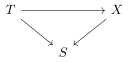• Morphisms:

\begin{align*} \operatorname{Mor}(T\to X, T'\to X) \mathrel{\vcenter{:}}= \left\{ \begin{array}{l} T\to T' \end{array} \,\, \middle\vert \begin{array}{l} (T \to T' \to X ) = g(T \to X) \\ g\in G(T) \\ G(T) \curvearrowright X(T) \end{array} \right\} .\end{align*}

A group scheme can alternatively be thought of as a functor with a factorization through $${\mathsf{Grp}}$$.

Show that for $$T\in {\mathsf{Sch}}$$, there is an equivalence \begin{align*} [X/G]^{\mathsf{pre}}(T) \xrightarrow{\sim} [X(T) / G(T)] ,\end{align*} where the left-hand side is a fibered category over $$T$$ and the right-hand side is a quotient groupoid.

### 2.3.1 Morphisms of Prestacks

A morphism of prestacks is a functor $${\mathfrak{X}}\xrightarrow{f} {\mathfrak{X}}'$$ such that there is a (strictly) commutative triangleHere we require a strict equality $$p_X(a) = p_Y(f(a))$$ for any $$a\in {\mathfrak{X}}$$

A 2-morphism $$\alpha$$ between morphisms $$f, g$$ is a natural transformation:such that for all $$a\in {\mathfrak{X}}$$, the following triangle $$\alpha_a\in \operatorname{Mor}_{{\mathfrak{X}}'}(f(a), g(a))$$ is a morphisms over $$\operatorname{id}_S$$ for any $$S\in \mathsf{C}$$:We define a category $$\operatorname{Mor}({\mathfrak{X}}, {\mathfrak{X}}')$$ by:

• Objects: morphisms of prestacks.
• Morphisms: 2-morphisms of prestacks.

Show that $$\operatorname{Mor}({\mathfrak{X}}, {\mathfrak{X}}')$$ is a groupoid.

A diagram is 2-commutative iff there exists a 2-morphism $$\alpha: g \circ f' \xrightarrow{\sim} f\circ g'$$ which is an isomorphism: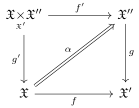An isomorphism of prestacks is a 1-isomorphism of prestacks $$f: {\mathfrak{X}}\to {\mathfrak{X}}'$$ along with 2-isomorphisms $$g\circ f \xrightarrow{\sim} \operatorname{id}_{{\mathfrak{X}}}$$ and $$f\circ g \xrightarrow{\sim} \operatorname{id}_{{\mathfrak{X}}'}$$.

Show that $${\mathfrak{X}}\to {\mathfrak{X}}'$$ is an isomorphism iff $${\mathfrak{X}}(S) \xrightarrow{\sim} {\mathfrak{X}}'(S)$$ is an isomorphism on all fibers.

If $${\mathfrak{X}}\in {\underset{ \mathsf{pre} } {\mathsf{St} } } {}_{/ {\mathsf{C}}}$$ is a prestack over $$\mathsf{C}$$, then for any $$S\in {\operatorname{Ob}}(\mathsf{C})$$, there is an equivalence of categories induced by the following functor: \begin{align*} \operatorname{Mor}(S, {\mathfrak{X}}) & \xrightarrow{\sim} {\mathfrak{X}}(S) \\ f &\mapsto f_S(\operatorname{id}_S ) .\end{align*}

For $$S\in {\mathsf{Sch}}$$, view $$S$$ as a prestack and consider a morphism $$f:S\to {\mathfrak{X}}$$. How is this specified? For all $$T\in {\mathsf{Sch}}$$, the objects of $$S_{/ {T}}$$ are morphisms \begin{align*} f_T: \operatorname{Mor}(T, S) \to {\mathfrak{X}}(T) \end{align*} and if $$T=S$$ this sends $$\operatorname{id}_S$$ to $$f_S(\operatorname{id}_S)\in {\mathfrak{X}}(S)$$.

What is the inverse? For $$a\in {\mathfrak{X}}(S)$$ and for each $$T \xrightarrow{g} S$$, choose a pullback $$g^* a$$. Then define $$f: S \to {\mathfrak{X}}$$ by \begin{align*} f_T: \operatorname{Mor}(T, S) &\to {\mathfrak{X}}(T) \\ g &\mapsto g^* a .\end{align*}

Define what this equivalence should do on morphisms.

Next time: fiber products of prestacks.

# 3 Bibliography

1. This leads to the notion of lax or pseudofunctors.↩︎